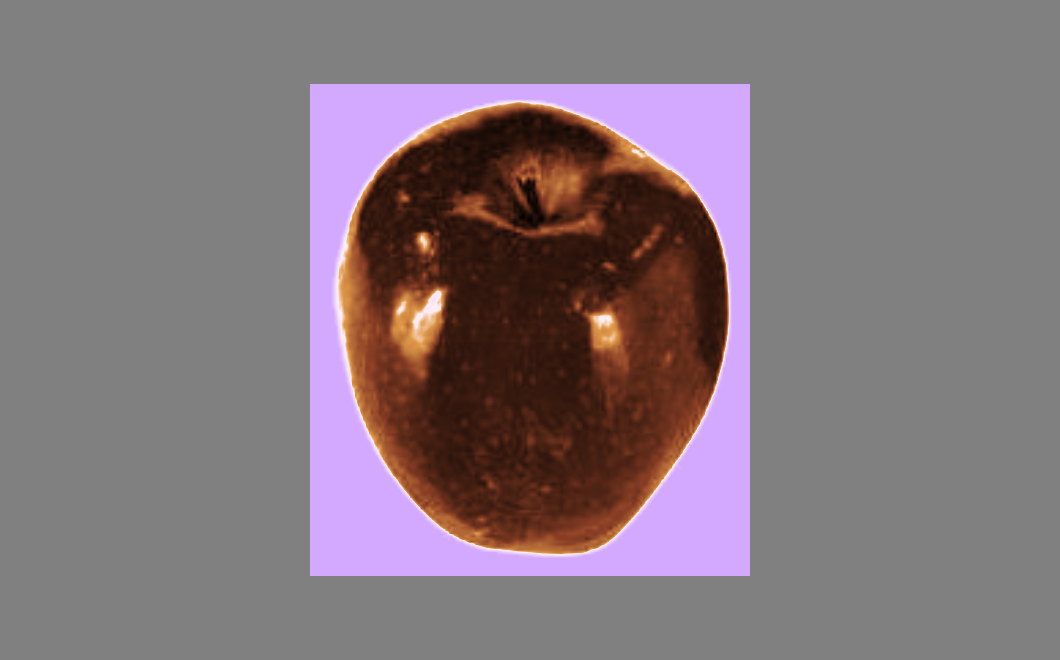# Visualize Parameter Space with Exhaustive Optimizer#

ITK optimizers are commonly used to select suitable values for various parameters, such as choosing how to transform a moving image to register with a fixed image. A variety of image metrics and transform classes are available to guide the optimization process, each of which may employ parameters unique to its own implementation. It is often useful to visualize how changes in parameters will impact the metric value and the optimization process.

The `ExhaustiveOptimizer` class exists to evaluate a metric over a windowed parameter space of fixed step size. This example shows how to use `ExhaustiveOptimizerv4` with the `MeanSquaresImageToImageMetricv4` metric and `Euler2DTransform` transform to survey performance over a parameter space and visualize the results with `matplotlib`.

```:
```
```import os
import sys
import itertools
from math import pi, sin, cos, sqrt
from urllib.request import urlretrieve

import matplotlib.pyplot as plt
import numpy as np

import itk
from itkwidgets import view

module_path = os.path.abspath(os.path.join("."))

if module_path not in sys.path:
sys.path.append(module_path)
```

## Get sample data to register#

In this example we seek to transform an image of an orange to overlay on the image of an apple. We will eventually use the `MeanSquaresImageToImageMetricv4` class to inform the optimizer about how the two images are related given the current parameter state. We can visualize the fixed and moving images with `ITKWidgets`.

```:
```
```fixed_img_path = "apple.jpg"
moving_img_path = "orange.jpg"
```
```:
```
```if not os.path.exists(fixed_img_path):
urlretrieve(url, fixed_img_path)
if not os.path.exists(moving_img_path):
urlretrieve(url, moving_img_path)
```
```:
```
```fixed_img = itk.imread(fixed_img_path, itk.F)
```
```:
```
```view(fixed_img)
``````:
```
```<itkwidgets.viewer.Viewer at 0x7f013463a640>
```
```:
```
```view(moving_img)
```